# How to Calculate and Solve for the Length and Area of a Pentagon | Nickzom Calculator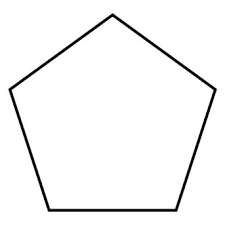The image above is a pentagon.

To compute the area of a pentagon, one essential parameter is needed and this parameter is length of side (a).

The formula for calculating the area of a pentagon:

A = a2(5(5 + 2√5) / 4

Where;
A = Area of the pentagon
a = length of side

Let’s solve an example;
Find the area of the pentagon when the length of side is 30 cm.

This implies that;
a = length of side = 30 cm

A = a2(5(5 + 2√5) / 4
A = 302(5(5 + 4.47) / 4
A = 900√(5(9.47) / 4
A = 900√47.36 / 4
A = 900√11.84
A = 900 x 3.44
A = 3096

Therefore, the area of the pentagon is 3096 cm2.

Calculating the length of side using the area of the pentagon.

a = √(4A / √5(5 + 2√5))

Where;
a = length of side
A = Area of the pentagon

Let’s solve an example;
Find the length of side with an area of 150 cm2.

This implies that;
A = Area of the pentagon = 150 cm2

a = √(4A / √5(5 + 2√5))
a = √(4 x 150 / √5(5 + 1.148))
a = √(600 / √5(6.148))
a = √(600 / √30.74)
a = √(600 / 5.54)
a = √108.30
a = 10.41

Therefore, the length of side is 10.41 cm.

Nickzom Calculator – The Calculator Encyclopedia is capable of calculating the area of a pentagon.

To get the answer and workings of the area of a pentagon using the Nickzom Calculator – The Calculator Encyclopedia. First, you need to obtain the app.

You can get this app via any of these means:

To get access to the professional version via web, you need to register and subscribe for NGN 1,500 per annum to have utter access to all functionalities.
You can also try the demo version via https://www.nickzom.org/calculator

Once, you have obtained the calculator encyclopedia app, proceed to the Calculator Map, then click on Mensuration under the Mathematics section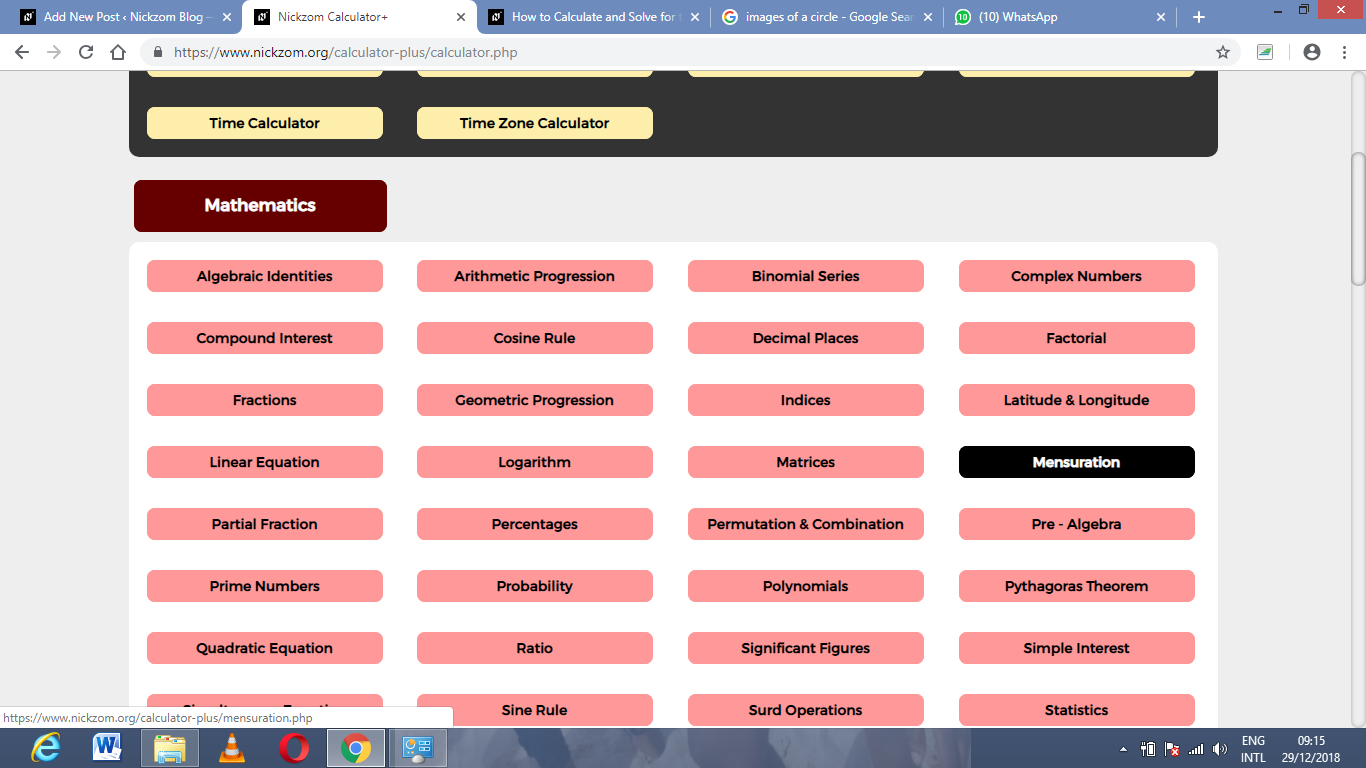Now, click on Area of a Pentagon under MensurationThe screenshot below displays the page or activity to enter your value, to get the answer for the area of a pentagon according to the respective parameter which is the length of side (a).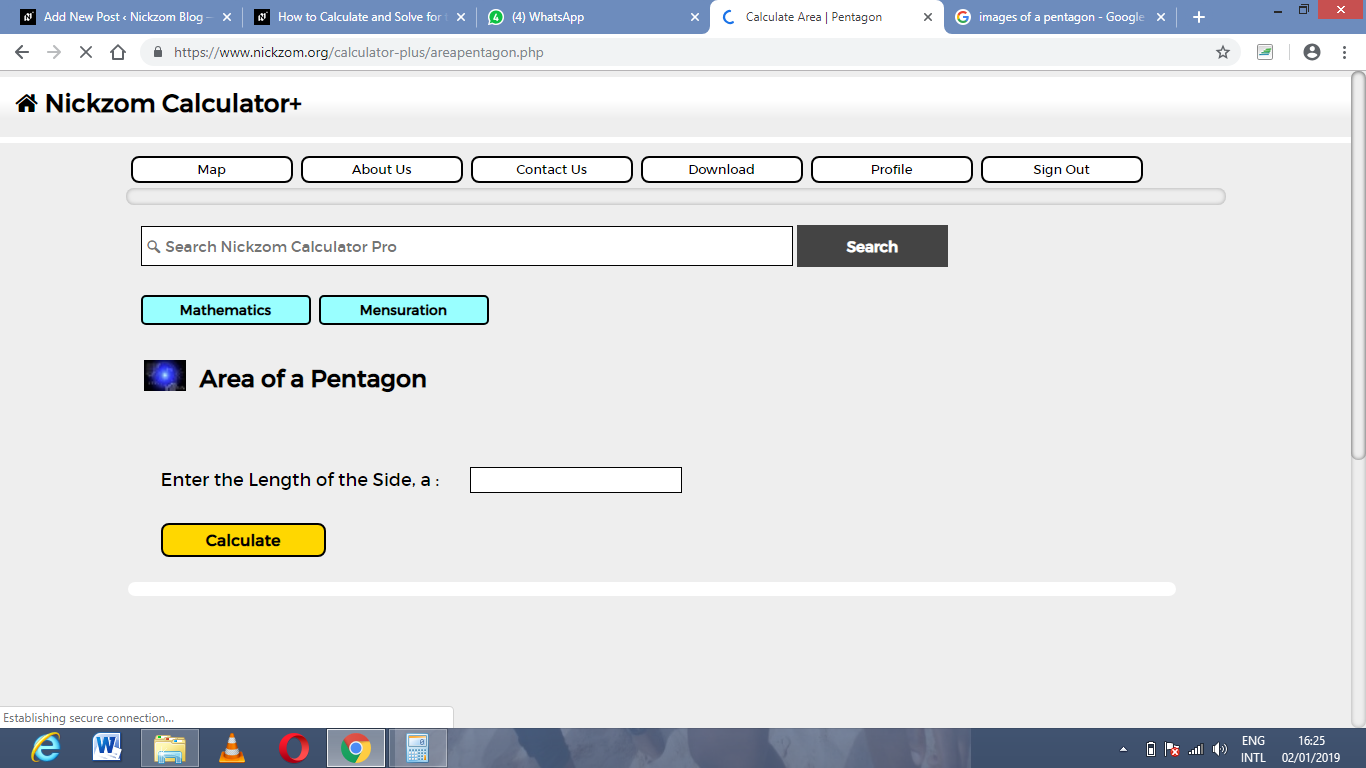Now, enter the values appropriately and accordingly for the parameter as required by the example above where the length of side (a) is 30 cm.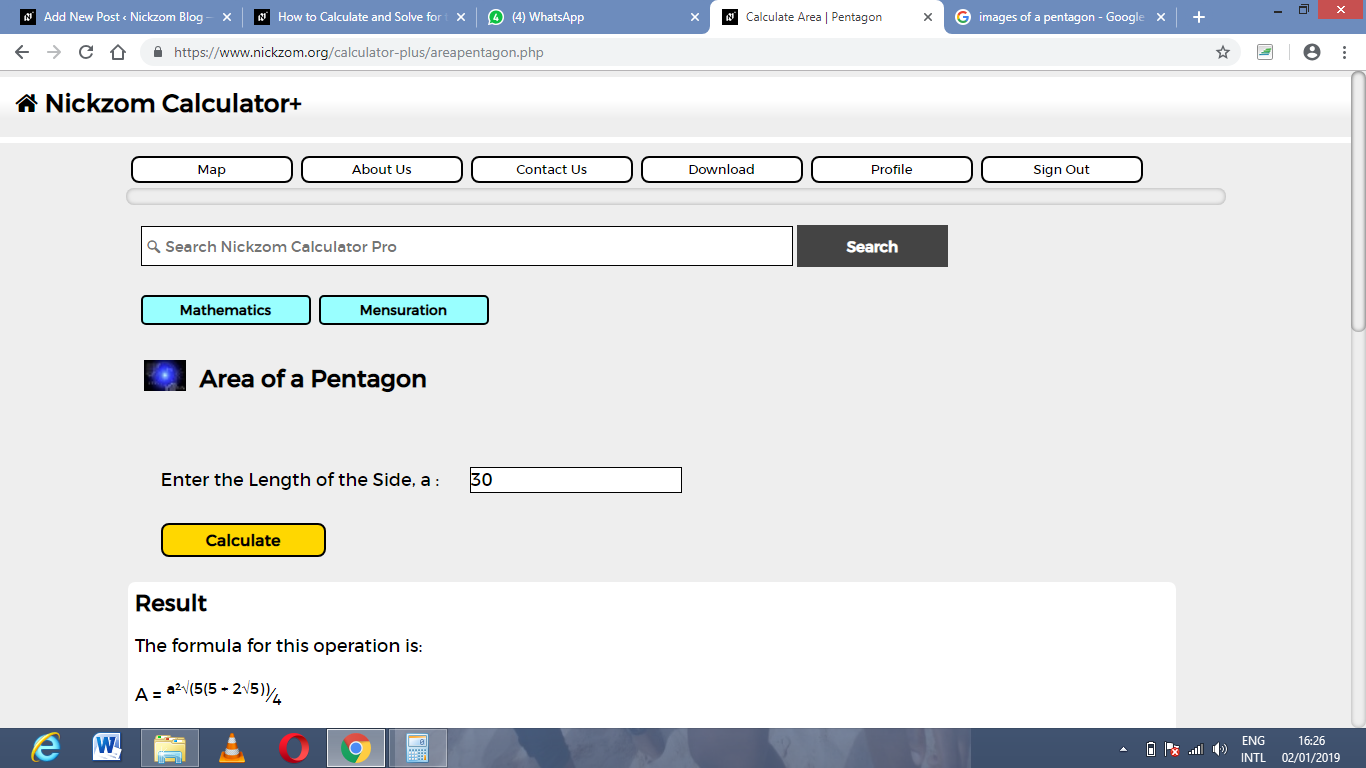Finally, Click on Calculate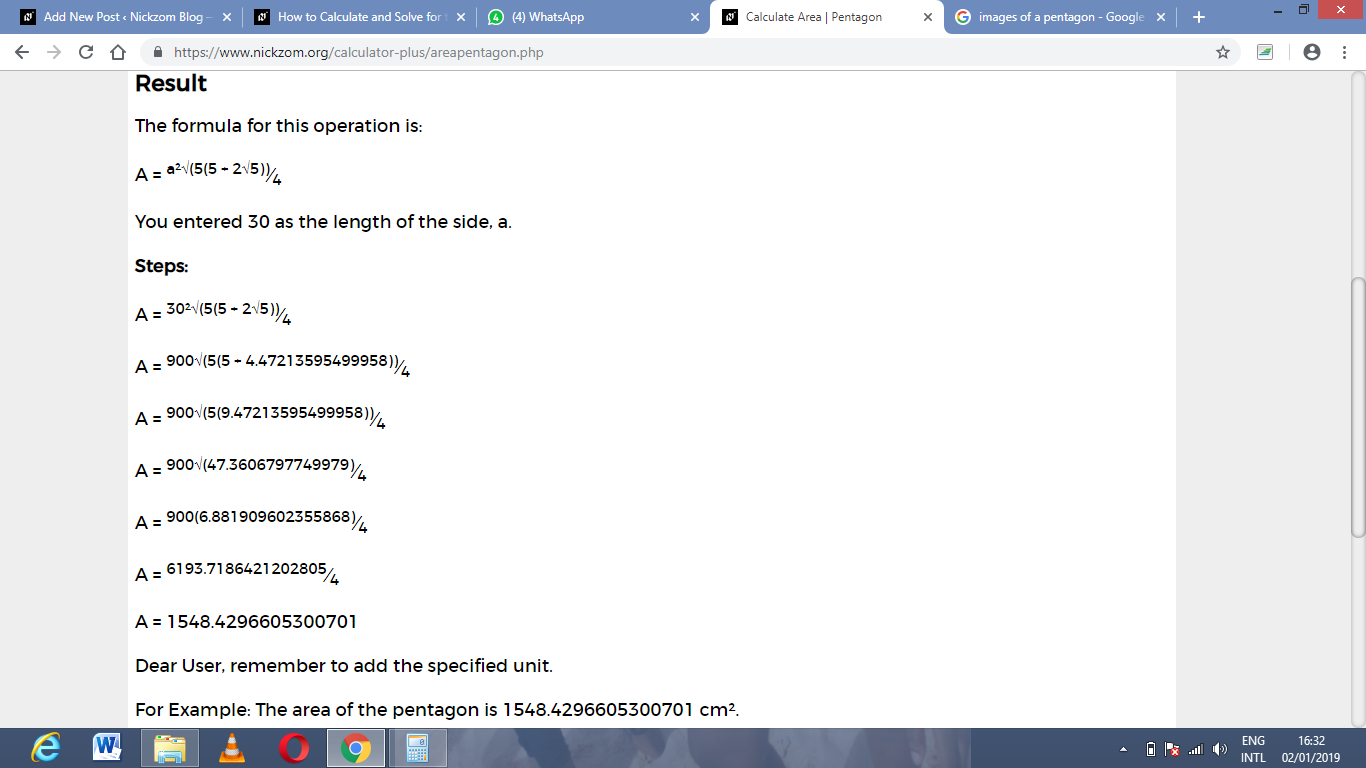As you can see from the screenshot above, Nickzom Calculator – The Calculator Encyclopedia solves for the area of a pentagon and presents the formula, workings and steps too.